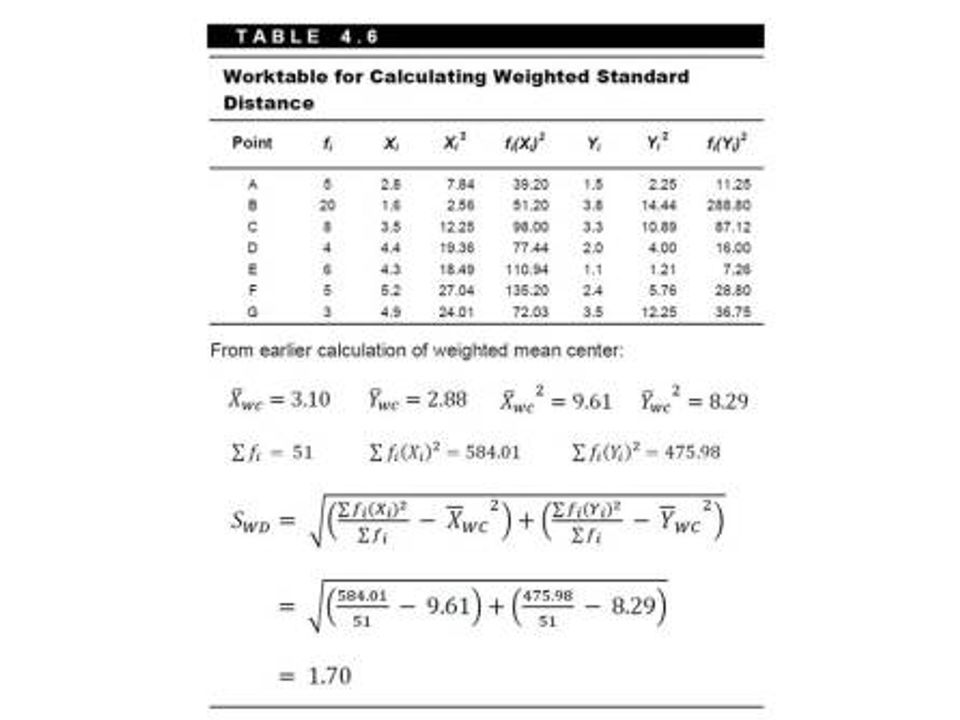top of page
Search

# Spatial is not special – Weighted Standard Distance

This post is part of a series entitled Spatial is not Special, where I will illustrate how spatial constructs in SQL provide us with a rich toolset for geographers doing spatial analysis.  To illustrate these concepts, I will be showing examples from my book Statistical Problem Solving in Geography.  Even though PostGRES, SQLServer, MySQL, spatialite, and Oracle all support spatial SQL, these examples will be presented using Manifold GIS.  The example dataset is a Manifold 8.0 .map file and can be found here.

As we continue to move through my book Statistical Problem Solving in Geography, we extend the computation of the standard distance to that of the weighted standard distance. Following our example from the book, we have an attribute column called f, which represents the frequency.  The computation is presented as:The SQL for computing the weighted standard distance is:

SELECT sqr(                        SUM(f*x^2)/(SELECT sum(f)                                             FROM Points)(SUM(x*f)/(SELECT sum(f)                                                                                  FROM Points))^2 + SUM(f*y^2)/(SELECT SUM(f)                                          FROM Points)(SUM(y*f)/(SELECT sum(f)                                                                                        FROM Points))^2                            ) FROM Points

This is almost identical to the standard distance computation, but instead of dividing by the number of features, we divide by the total frequency. We also subtract the weighted mean center coordinates, instead of simply the mean center.  And finally, we multiply each coordinate by the frequency. Once again, the proper placement of parentheses allow us to wrap that calculation inside the square root function. I’ve colored the parentheses to help understand which ones correspond to one another – hopefully the colors are more helpful than distracting.  This is what is so nice about having a worked example – putting this into SQL took me a little time because of the parenthesis issue.

So, this gets us the weighted standard distance. LIke the previous example of weighted mean center, we can wrap the query in the buffer function to create a geometry of the weighted standard distance centered on the weighted mean center.

SELECT buffer(newpoint((SUM(x*f)/(SELECT sum(f) FROM Points))^2, (SUM(y*f)/(SELECT sum(f) FROM Points))^2),                                           sqr                                              (SUM(f*x^2)/(SELECT sum(f)                                              FROM Points) – (SUM(x*f)/(SELECT sum(f)                                                                                           FROM Points))^2                                              + SUM(f*y^2)/(SELECT SUM(f)                                                                      FROM Points) – (SUM(y*f)/(SELECT sum(f)                                                                                                   FROM Points))^2))         FROM Points

Remember from earlier posts that the buffer function requires a geometry and a distance [buffer([geometry],distance)]. So, for the [geometry] field, we are passing our mean center, and for our distance, we are passing our standard distance. Again, the hardest thing about this is getting the parentheses correct!!!  Always work with a small example so you can make sure your answers are correct – this will scale just fine once you have it working.

Hopefully, with the gray text, you can see how easy it is to take the results from the standard distance query along with the mean center geometry, and just insert it into the buffer function.